GRE Subject Test: Biochemistry, Cell, and Molecular Biology : Help with Quantification Techniques

Example Questions

Example Question #12 : Analytical Chemistry

A researcher performs a Bradford assay to determine the quantity of an unknown protein in his sample. The standard protein returns absorbance values of 0.101, 0.204, 0.302, 0.405 for the respective quantities of 10ug, 20ug, 30ug, and 40ug of protein. The unknown sample returns an absorbance value of 0.265. What is the quantity of protein in the unknown sample?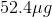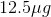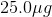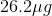Explanation:

For this problem we need to determine the equation of our standard curve. This can be done by creating a graph from the data points and determining the slope.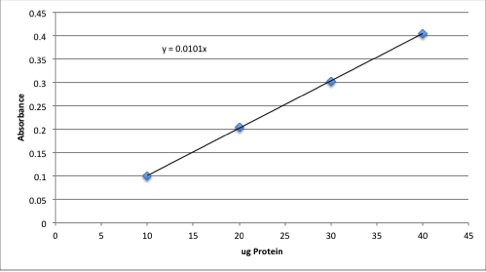Assuming that a sample of zero concentration will also have zero absorbance, we can find the equation for the line generated by finding the slope.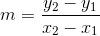Pick two points on the line to find the slope. We will use (0,0) and (40,0.405).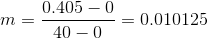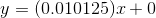Use this equation and the absorbance given in the question to find the concentration of the unknown sample.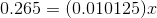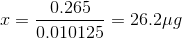Example Question #1 : Help With Quantification Techniques

Which of the following techniques would help quantify the amount of protein in a sample?

Southern blot

Coimmunoprecipitation

qPCR

Explanation:

Bradford assays use shifts in absorption ranges to identify the quantity of a protein in a sample. The technique uses Coomassie blue dye (Bradford reagent) and spectrophotometry to analyze protein concentrations.

qPCR is a procedure that measures the products made from a PCR reaction in real time, and is a helpful tool for studying gene expression. Coimmunoprecipitation is a technique that will isolate a specific protein or protein complex by using an antibody specific to that protein; however, this technique will not quantify a sample without further analysis. Southern blots are used to detect specific DNA sequences in a sample.

Example Question #2 : Help With Quantification Techniques

A researcher wants to know the exact concentration of protein in his sample. He estimates the amount of protein to be between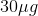and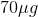. Which of the following sets of known protein amounts should he use to set up a standard curve for a Bradford assay?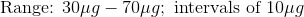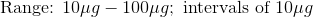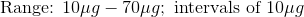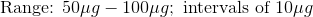Explanation:

Because he is unaware of exactly where the concentration lies, it would be best to test a few samples before and after his predicted range. This will allow the researcher to ensure that his curve is linear and that his sample fits accurately somewhere along the curve. If his sample falls outside of the range he tested, he will not be able to use his standard curve to accurately predict the concentration of his sample.

Example Question #4 : Help With Quantification Techniques

A researcher wants to measure the purity of DNA using a spectrophotometer. What wavelength(s) should he/she consider?

A280

The ratio of A160/A780

A260

The ratio of A260/A280

The ratio of A280/A260

The ratio of A260/A280

Explanation:

The correct answer is the ratio of A260/A280. DNA absorbs light most strongly at 260nm and is used to determine the concentration of the DNA solution. Contaminants such as aromatic amino acids present in proteins are absorbed at 280nm, and as such, taking the ratio of A260/A280 will indicate the purity of the DNA sample. Typically, a A260/A280 ratio of 1.7-2 is indicative of good quality DNA.

Example Question #3 : Help With Quantification Techniques

What is the purpose of a Bradford Assay?

To quantify DNA concentration in a sample

To quantify RNA concentration in a sample

To determine the percentage of cells undergoing apoptosis

To quantify protein concentration in a sample

To determine the percentage of viable cell culture cells in a population